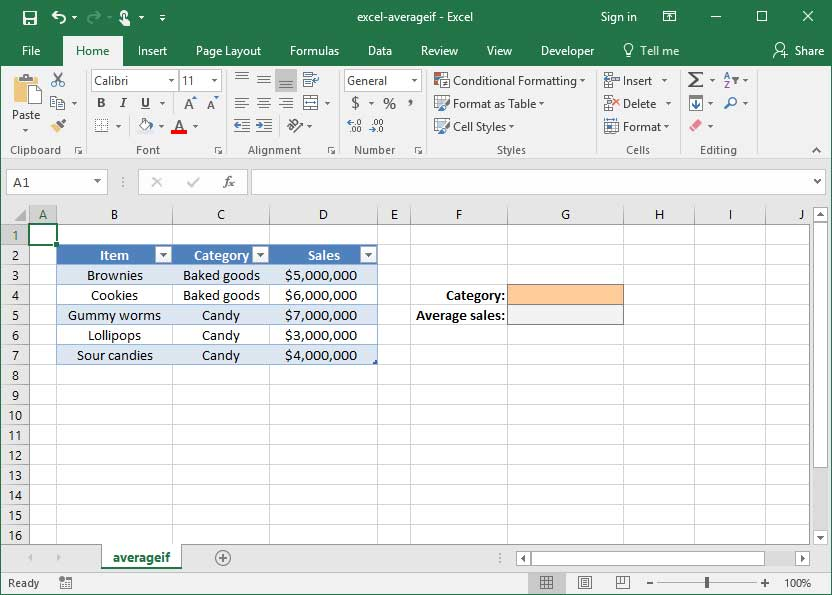# The AVERAGEIF function

Once you've learned about the SUMIF and COUNTIF functions, there's one more logical-mathematical function combination that's important to learn: AVERAGEIF. Like its cousins, AVERAGEIF takes the AVERAGE of a range of numbers conditional upon a particular criteria being met.

Since the AVERAGEIF syntax is very similar to the syntax for SUMIF and COUNTIF, we won't cover it in as granular detail here. Rather, we'll provide a short example to demonstrate its use.

If you aren't yet familiar with the SUMIF formula, take a minute to check out our SUMIF tutorial before reading ahead. The fundamentals of both functions are the same, and our tutorial on SUMIF provides even more practical examples.

## Defining the problem

Take a look at the spreadsheet below, which lists SnackWorld sales by item and category.Let's say we want to find the average sales of items in the Baked Goods category. We could do this manually — which is fine for a small table like this one — but that becomes difficult when we have a table that contains a lot more data.

That's where AVERAGEIF comes in. It'll allow us to count the number of occurences of a particular phrase within a range.

## AVERAGEIF in action

The formula for AVERAGEIF is as follows:

`=AVERAGEIF(range, criteria, average_range (optional))`

Using the formula is simple: simply specify the range that will be tested against the given criteria; the criteria against which you'd like to test; and the average_range of values to average. Let's apply the formula to the sample spreadsheet shown above.`=AVERAGEIF(C3:C7, "="&G4, D3:D7)Output: \$5,500,000`

Note that in this case, we're using the longform version of criteria"="&G4. Like SUMIF, AVERAGE also has a shortcut when our criteria contains =: in the formula above, we could replace "="&G4 with a simple G4, like so:

`=AVERAGEIF(C3:C7, G4, D3:D7)Output: \$5,500,000`

## Save an hour of work a day with these 5 advanced Excel tricks

Work smarter, not harder. Sign up for our 5-day mini-course to receive must-learn lessons on getting Excel to do your work for you.

• How to create beautiful table formatting instantly...
• Why to rethink the way you do VLOOKUPs...
• Plus, we'll reveal why you shouldn't use PivotTables and what to use instead...